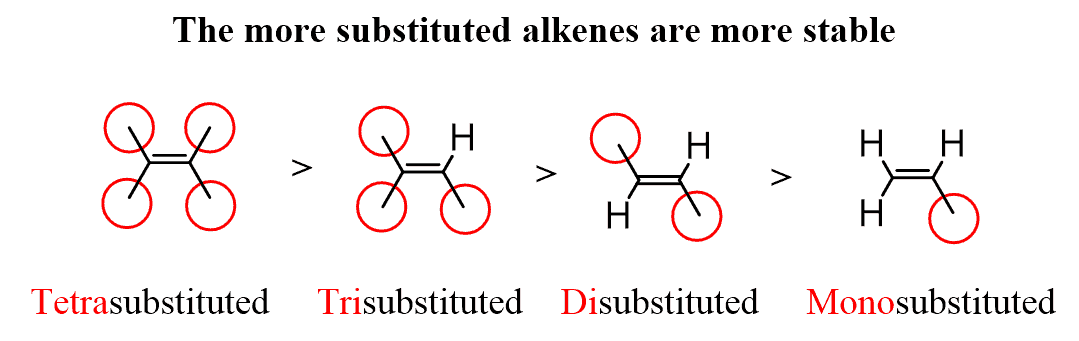# Role of alcoholic potassium hydroxide in an E2 reaction

Which of the following compound will give least substituted alkene as major product with alcoholic $$\ce{KOH}$$.

The options are:

I am confused between options 1 and 3. Both seem to give more substituted alkene as the major product. Does alcoholic $$\ce{KOH}$$ always mean it is an E2 elimination?

What is the mechanism here?

• Comments are not for extended discussion; this conversation has been moved to chat. – andselisk Jul 22 at 11:59

Haloalkanes react with alc. $$\ce{KOH}$$ mainly via $$E_2$$ mechanism. But, in some cases, where $$\beta-\ce{H}$$ isn't present, it proceeds via $$E_1$$, as in case of $$(4)$$ one. Let's analyze each option,

# Option $$(1)$$:

Firstly, the $$\ce{-Cl}$$ departs, and then the base picks up a $$\beta-\ce{H}$$ from the terminal methyl group (i.e., $$\ce{C-5}$$), resulting in mono- substituted alkene (Hofmann product as major). Steric factors are significant in selectivity of $$\beta-\ce{H}$$. Therefore, Zaitsev product will be formed in very minor concentrations, bcoz $$\ce{-C(CH3)2Ph}$$ is more bulkier substituent than $$\ce{^tBu}$$.

# Option $$(3)$$:

This one is similar to $$(1)$$ case, except for the fact that due to less steric hindrance, it will give di- substituted alkene (Zaitsev product).

Option $$(2)$$: It's clear that this molecule will form a tri- substituted alkene.

Option $$(4)$$: As hinted above, it will proceed through $$E_1$$, resulting in a tri- substituted alkene.

Different levels of substitution are shown below, (source: www.chemistrysteps.com)• – Safdar Jul 23 at 6:35